Select Page

# Piezoelectric Fundamentals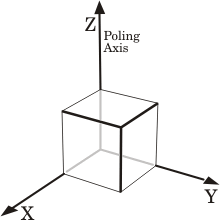The piezoelectric effect involves the interaction between the electrical and mechanical behaviour of a material. The material constants below describe that interaction.
The direction of polarization is generally designated as the z-axis of an orthogonal crystallographic system.
Material characteristics depend on the direction of the applied electric field, displacement, stress, and strain. Superscripts and subscripts are used to indicate direction.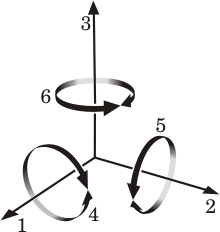The axes x,y and z are respectively represented as 1, 2 and 3 directions and the shear about these axes are represented as 4, 5 and 6. Planar modes are expressed with a subscript p.

The first subscript gives the direction of the electrical field associated with the voltage applied or the charge produced. The second subscript gives the direction of mechanical stress or strain. Superscripts indicate a constant mechanical or electrical boundary condition.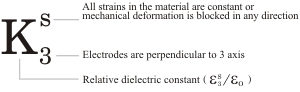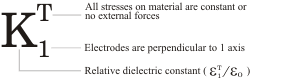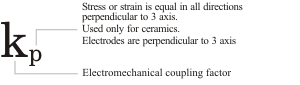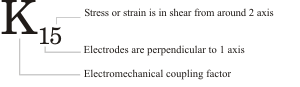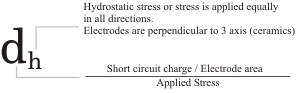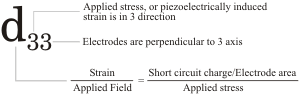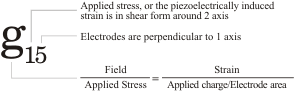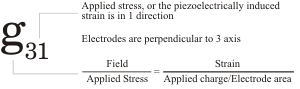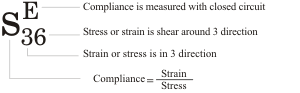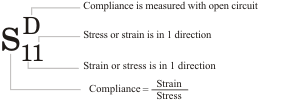## Density

Density, ρ, of a material is expressed as the ratio of the mass of a body to its volume. Density can be measured using a balance and a micrometer. A buoyancy technique provides better accuracy and has the advantage that measurements can be made on any shape.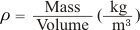## Curie Temperature

The crystal structure of a material changes at the Curie temperature from piezoelectric (non-symmetrical) to a non-piezoelectric (symmetrical) form, and the material loses its piezoelectric properties. This phase change is accompanied by a peak in the dielectric constant at that temperature. The Curie temperature, Tc, is expressed in degrees Celsius.

## Dielectric Constant (Relative Permittivity)

The dielectric constant is defined as the ratio of the permittivity of the material to the permittivity of free space. This is generally measured well below the mechanical resonance. The dielectric constant is derived from the static capacitance measurements at 1 kHz using a standard impedance bridge. The relations are expressed as shown in Figure 2 by the associated equation.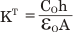where:
KT: relative dielectric constant of the material
εo: relative permitivity of free space (8.854 x 10-12 F/m)
h: distance between electrodes (m)
A: area of the electrodes (m2)
Co: measured capacitance at 1kHz (F)

Figure 2: Notation for Relative Permittivity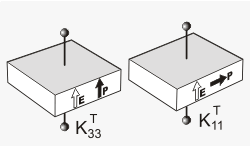## Dielectric Loss Factor (Loss Tangent)

The dielectric loss factor, also known as dissipation factor, is defined as the tangent of the loss angle (Tan d). The loss factor represents the ratio of resistance to reactance of a parallel equivalent circuit of the ceramic element, Figure 3. The dielectric constant (K) and loss factor (Tan d) can be measured using a standard impedance bridge or an impedance analyzer, both of which provide a direct reading. Measurements are generally carried out at 1 kHz to measure static (away from mechanical resonance) parameters at low applied electric field.

Figure 3: Piezoelectric Ceramic Equivalent Circuit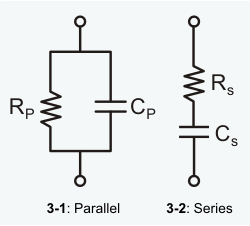## Mechanical Quality Factor

The mechanical Q (also referred to as QM) is the ratio of the reactance to the resistance in the series equivalent circuit representing the piezoelectric resonator (Figure 3). The QM is also related to the sharpness of the resonance frequency.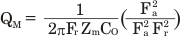where:
Fr: resonance frequency (Hz)
Fa: anti-resonance frequency (Hz)
Zm: impedance at Fr (ohm)
C0: static capacitance (Farad)

Alternatively QM can also be determined using the equation: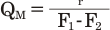where:
F1, F2 are points on the impedance vs. frequency curve -3dB from the resonance frequency Fr.

## Frequency Constants

The frequency constant, N, is the product of the resonance frequency and the linear dimension governing the resonance.

N1=Fr D (Hz.m) Radial Mode Disc
N2=Fr l (Hz.m) Length Mode Plate
N3=Fr l (Hz.m) Length Mode Cylinder
N4=Fr h (Hz.m) Thickness Mode Disc, Plate
N5=Fr h (Hz.m) Shear Mode Plate

## Piezoelectric Coupling Coefficient

The coupling coefficient (sometimes referred to as the electromechanical coupling coefficient) is defined as the ratio of the mechanical energy accumulated in response to an electrical input or vice versa. The piezoelectric coupling coefficient can be expressed in the following equation: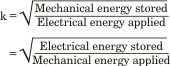The coupling coefficients can be calculated for the various modes of vibration from the following equations: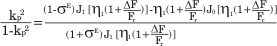where:
Jo: Bessel function of the first kind and zero order.
J1: Bessel function of the first kind and first order.
σE: Poisson’ Ratio.
η1: Lowest positive root of ( 1+σE) J1 η = ηJ0 (η)

It has been assumed that σE = 0.31 for lead zirconate titanate and η1 = 2.05.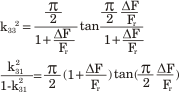where ΔF = Fa-Fr (Hz).

Another parameter, keff, is frequently used to express the effective coupling coefficient of an arbitrary resonator, either at fundamental resonance or at any overtone, and is expressed as follows: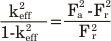## Piezoelectric Charge Coefficients (d-Constant)

The piezoelectric charge coefficient is the ratio between the electric charge generated per unit area and an applied force and is expressed in Coulomb/Newton (C/N).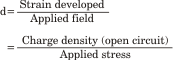The d constants are calculated from the equation: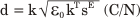where:
k: electro-mechanical coupling coefficient
KT: relative dielectric constant
sE: compliance (10-12m2/N)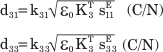## Piezoelectric Voltage Coefficient (g-Constant)

The piezoelectric voltage coefficient is the ratio of the electric field produced to the mechanical stress applied and is expressed as Volt.meter/Newton (Vm/N).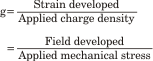The g-constants are calculated from the equation: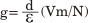where:
d: charge density
ε: relative permittivity

## Elastic Constants

Young’s modulus describes the mechanical stiffness properties and is expressed as the ratio of stress to strain. In a piezoelectric material, mechanical stress produces an electrical response which opposes the resultant strain. The value of the Young’s modulus depends on the direction of stress and strain and the electrical conditions. The inverse of Young’s modulus, Y, is the elastic compliance, s.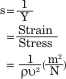where:
ρ: density (kg/m3)
ν: sonic velocity ( = 2pFl ) (m/sec)
l: length of the element (m)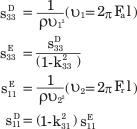## Ageing Rate

The ageing rate of a piezoelectric ceramic is a measure of how certain material parameters vary as a function of time (age). The most important parameters that age with time are the dielectric constant, frequency constants and the resonant frequency. The ageing of ceramics has a logarithmic function with time.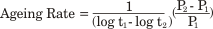where:
t1, t2: number of days after polarization
P1, P2: measured parameters such as capacitance, resonance frequency, etc.